# Rectangle facts for kids

Kids Encyclopedia Facts

In geometry, a rectangle is a shape with four sides and four corners. The corners are all right angles. It follows that the pairs of sides opposite each other must be parallel and of the same length.

People make many rectangular things, including most tables, boxes, books, and papers. The word comes from Latin words meaning "right" and angle".

A rectangle whose four sides have the same length is called a square.

## Formulas

If a rectangle has length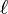$\ell$ and width w, then:

• It has area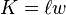$K = \ell w$.
• it has perimeter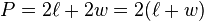$P = 2\ell + 2w = 2(\ell + w)\,$.
• Each diagonal has length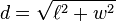$d=\sqrt{\ell^2 + w^2}$.
• it has an aspect ratio of$\ell$ : w.
• When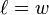$\ell = w\,$, the rectangle is a square.
• When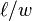$\ell / w$ is equal to the golden ratio, the rectangle is called a golden rectangle.

## Images for kidsRectangle Facts for Kids. Kiddle Encyclopedia.